Open in App
Not now

# ∈-NFA of Regular Language L = (01 + 2*)1

• Last Updated : 19 Jan, 2021

Epsilon NFA is a part of Finite Automata. ∈ is a symbol that represents empty inputs. ∈-NFA is the representation that allows an automaton to change its state without input. ∈-Non-Deterministic Finite Automata has a different transition function than regular NFA. Here is the formal definition of ∈-NFA.

```∈-NFA is defined in 5 tuple representation {Q, q0, Σ, δ, F} where
Q is the set of all states,
q0 is the initial state,
Σ is the set of input symbols,
δ is the transition function which is δ:Q × (Σ∪∈)->2Q and
F is the set of final states.```

Simple rules for construction of ∈-NFA :

`∈-NFA for a+ :`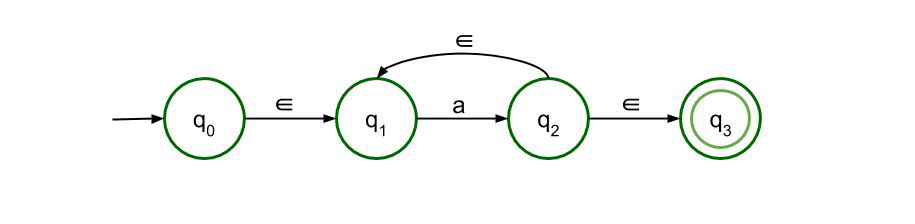Here, ‘a+’ means that there must be at least one ‘a’ in the input expression for it to be acceptable. It is preceded and succeeded by epsilon because the expression may or may not contain anything else at all. There is epsilon feedback from state q2 to q1 so that there can be more than one ‘a’ in the expression.

`∈-NFA for a* :`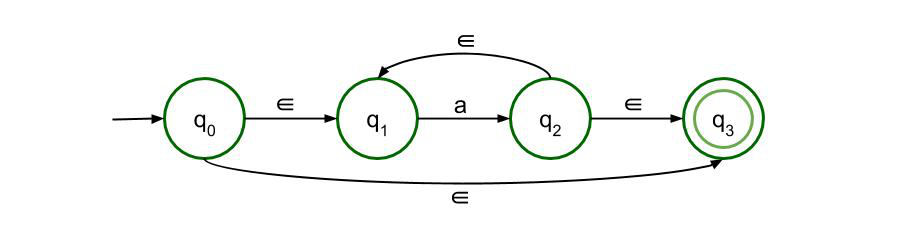Here, ‘a*’ means that there can be any number of ‘a’ in the expression, including 0. The previous structure is just modified a bit so that even if there is no input symbol, i.e. if the input symbol is null, then also the expression is valid.

`∈-NFA for a+b :`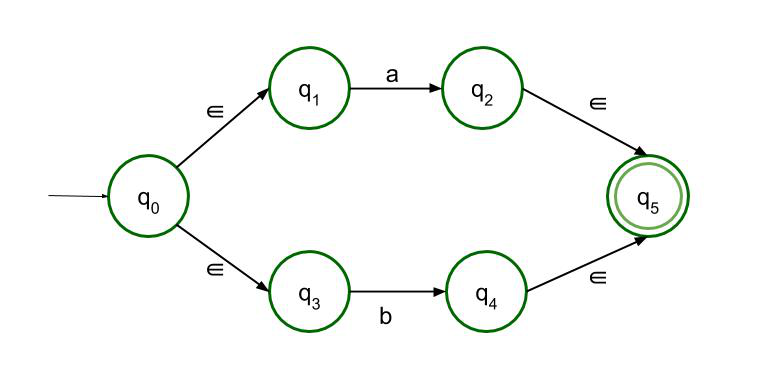This structure accepts either a or b as input. So there are two paths, both of which lead to the final state.

`∈-NFA for ab :`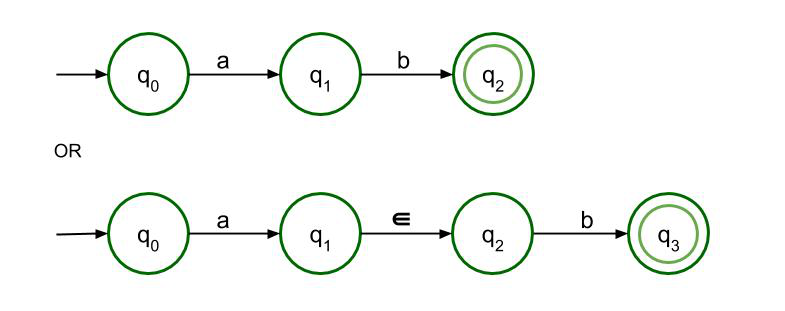For concatenation, a must be followed by b. Only then it can reach the final state. Both structures are allowed here but as it is ∈-NFA so the second structure is recommended.

∈-NFA for L = (01+2*)1 :

Following the above-mentioned rules, ∈-NFA of Regular Language L = (01+2*)1 is to be constructed.

L = (01+2*)1 can be divided into two parts. The first part is (01+2*) and the second part is 1. Since they are concatenated, so they are to be linearly connected with the help of the fourth rule. Now the first part can be further divided into two parts – 01 and 2*.  01 can be drawn with the help of the fourth rule considering a = 0 and b = 1. 2* can be drawn with the help of the second rule considering a = 2. Now since 01 and 2* are connected with a ‘+’ sign, there will be two paths from the first node, both of which will reach the penultimate node. This structure when followed by a ‘1’ gives us the final ∈-NFA of the above-mentioned language.

The Final ∈-NFA will be :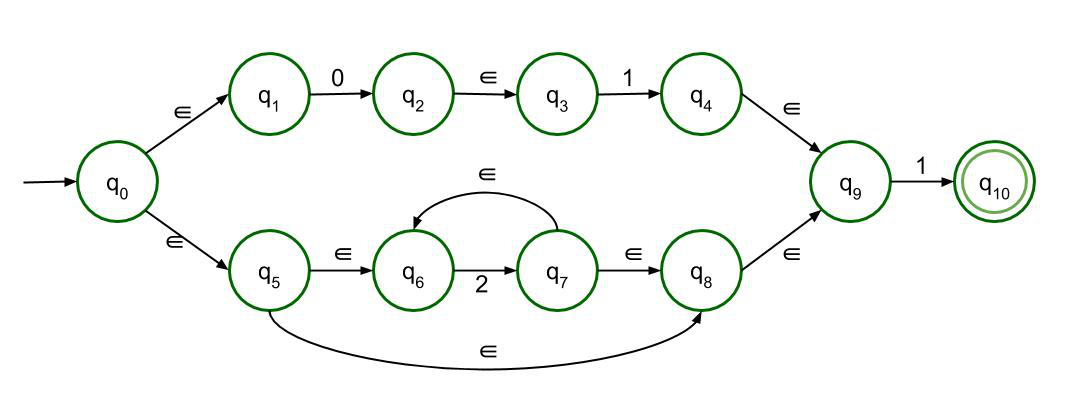My Personal Notes arrow_drop_up